Short Notes: Structure of the Atom

# Short Notes: Structure of the Atom - Notes | Study Science Class 9 - Class 9

 Table of contentsAtomic StructureThomson's model of an AtomRutherford's Model of an AtomBohr's Model of AtomDistribution of Electrons in Different OrbitsValencyAtomic NumberMass NumberIsotopesIsobars1 Crore+ students have signed up on EduRev. Have you?

The structure of an atom comprises protons, neutrons and electrons. These basic components provide the mass and charge of the atoms.

Atomic Structure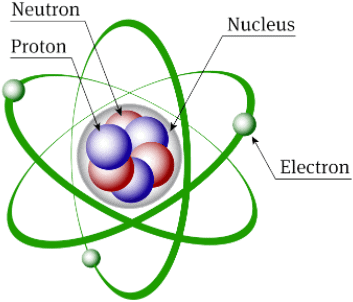• John Dalton assumed that the atom is indivisible.
• In 1866 E. Goldstein discovered the presence of new radiations in a gas discharge tube and called them canal rays. These rays were positively charged radiations which led to the discovery of a sub-atomic particle called proton.
•  In 1897 J.J. Thomson discovered the sub-atomic particle - the electron with a negative charge.
• The neutron was discovered by Chadwick. Neutrons have no charge.
• Table: Properties of Electrons, Neutrons and Protons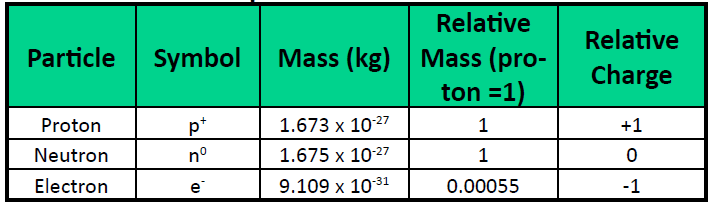Question for Short Notes: Structure of the Atom
Try yourself:Question 1: Which component of atom has a postive charge?

Thomson's model of an Atom

• In the sphere of protons, electrons are distributed.
• The total positice charge in an atom equal to the total negative charge.
• Atom is electrically neutral.
• Also known as the plum pudding model.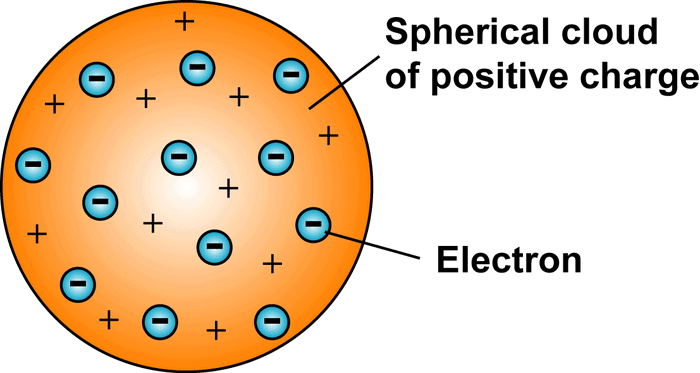Plum Pudding Model

Rutherford's Model of an Atom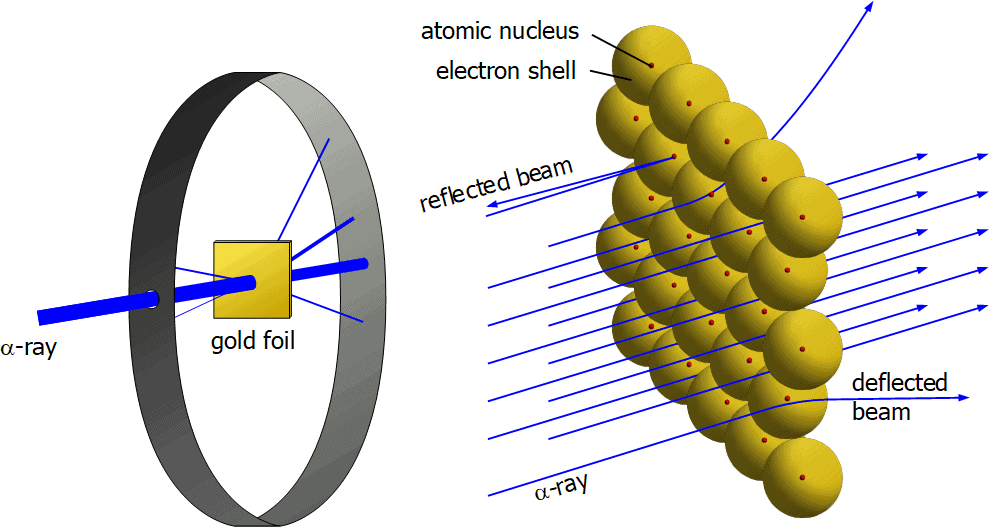Rutherford's Experiment

• α-Particles: (+2 charge and 4 mass) when fast-moving α-particles are bombarded on very thin gold foil, the following observations were made:
• Most of the α-particles passed straight through the gold foil.
• Some of the α-particles were deflected by the foil by small angles.
• One out of 12000 particles appeared to rebound.

• Most of the space inside the atom is empty because most of the α-particles passed through the gold foil.
• Very few particles were deflected from their path because the +ve charge of the atom occupies very little space.
• A very small fraction of α-particles were rebounded back, shows all + ve charge and mass of the gold atoms were concentrated in a very small volume within the atom.
• The radius of the nucleus calculated was 105 times less than the radius of the atom.
• Nuclear Model of an Atom given by Rutherford
(i) Centre → +ve charge → called nucleus. All mass resides in nucleus.
(ii) Electrons → revolve around the nucleus in orbits.
(iii) Size of nucleus is very small as compared to the size of the atoms.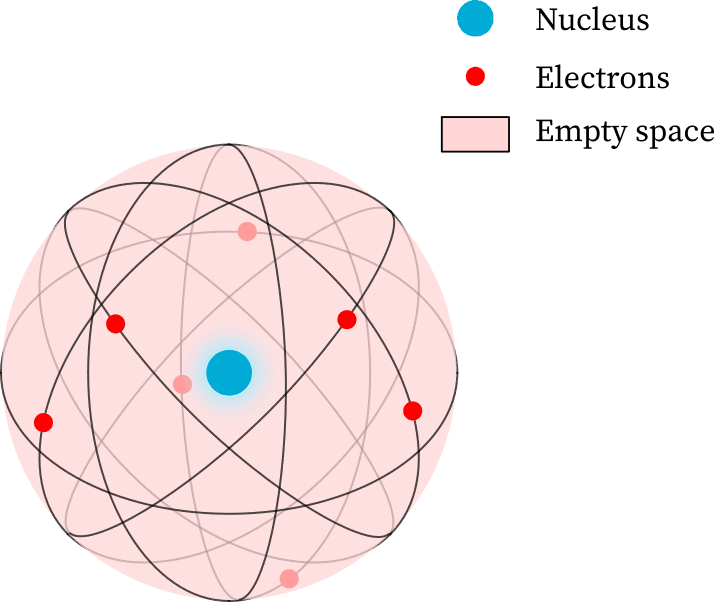Rutherford's Nuclear Model of Atom

Drawbacks of Rutherford's model of the atom

• When an electron undergoes acceleration, it radiates energy. Thus revolving electron would lose energy and finally fall into the nucleus
• Due to this atom should be highly unstable and hence matter would not exist in the form that we know.
• But we know that atoms are quite stable.

Question for Short Notes: Structure of the Atom
Try yourself:Question 2: Rutherford’s ‘alpha (α) particles scattering experiment’ resulted in the discovery of

Bohr's Model of Atom
Postulates of Neil Bohr

• Only special orbits known as discrete orbits of electrons are allowed inside the atom.
• While revolving in discrete orbits the electrons do not radiate energy.
• These orbits are called energy levels.
• Orbits or shells are represented by K, L, M, N or the numbers, n = 1, 2, 3, 4, .......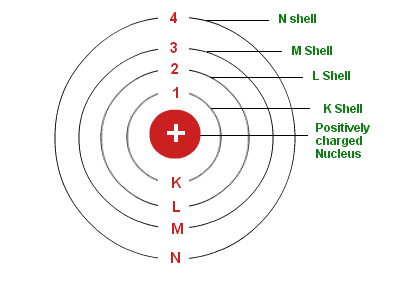Bohr's Model

Drawbacks of Bohr's Model of Atom

• It violates the Heisenberg Uncertainty Principle.
• It could not explain the spectra obtained from larger atoms.

Distribution of Electrons in Different Orbits

Rules

• Maximum number of electrons present in a shell is given by 2n2 (n = shell number)

Example: maximum number of electrons present in n = 1 are

n = 1 (K shell)

2(1)2 = 2 electrons

• The maximum number of electrons that can be accommodated in the outermost orbit is 8.
• Electrons are not accommodated in a given shell unless the inner shells are completely filled.

Valency

• The combining capacity of an atom is called its valency.
• The number of bonds that an atom can form as part of a compound is expressed by the valency of the element.
• Valence electrons are those electrons that are present in the outermost orbit of the atom.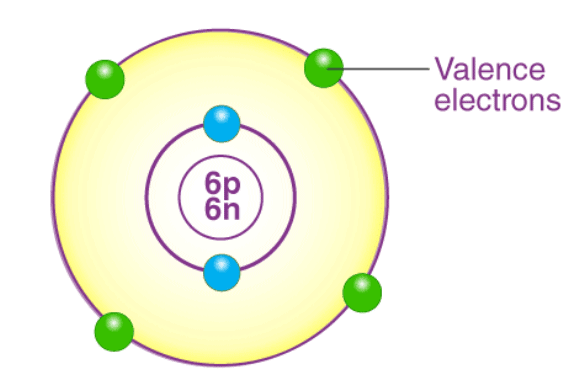Atomic Number

• The total number of protons in the nucleus of an atom gives us the atomic number of that atom.
• It is represented with the letter ‘Z.’

Mass Number

• The number of protons and neutrons combined to give us the mass number of an atom.
• Mass Number is also called Atomic Mass.
• It is represented using the letter ‘A.’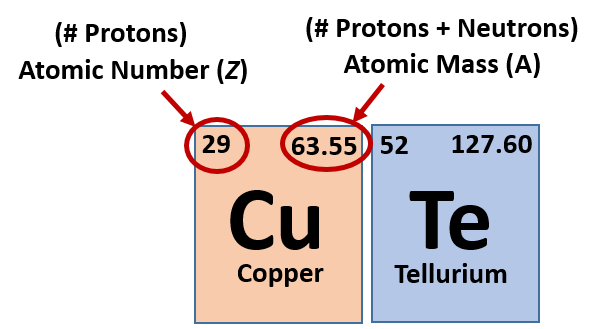An element is represented by AXZ, where Z is atomic number which is also equal to number of proton, A is mass number and X is symbol of the element. Mass number (A) = Number of protons (Z) + Number of neutrons.

Isotopes

• Atoms of the same element with the same atomic number but a different mass number, are called isotopes.
• Chemical properties → same
• Physical properties → different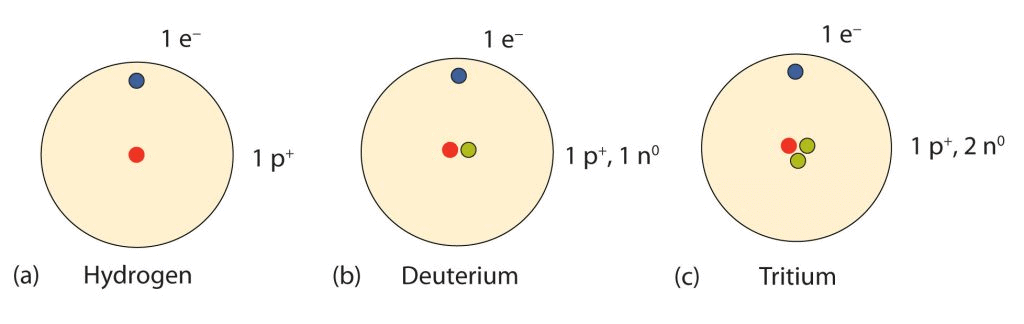Isotopes of Hydrogen
Applications of Isotopes:
(a) An isotope of Uranium is used as fuel in nuclear reactors.
(b) An isotope of Cobalt is used in the treatment of cancer.
(c) An isotope of Iodine is used in the treatment of goitre.

Question for Short Notes: Structure of the Atom
Try yourself:Question 3: The number of electrons in an element X is 15 and the number of neutrons is 16. Which of the following is the correct representation of the element?

Isobars

• Atoms of different elements with the same mass number but different atomic numbers are called isobars.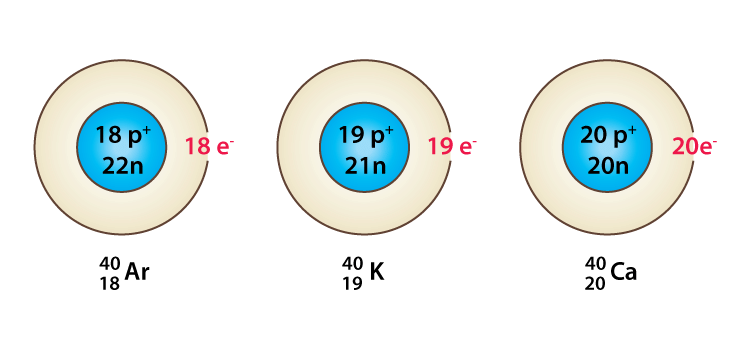Examples of Isobars

Some Practice Questions:

Ques. How hydrogen atom is different from atoms of all other elements?

Ans. All the atoms are made up of three subatomic particles: electrons, protons and neutrons. Hydrogen atom is made up of only one electron and one proton. It does not contain any neutron. So, it is different from atoms of all other elements.

Ques. What is mass number?

Ans. Mass number of an element is the sum of number of protons and neutrons present in the atom of the element.

Mass number = No. of protons + No. of neutrons.

Example: Hydrogen atom has 1 proton but 0 neutron thus the mass number of H is 1.

The mass number of an element is denoted by the letter A. Protons and neutrons present in a nucleus, together known as nucleons.

Hence, Mass number = Number of nucleons.

Ques. What are the general features of isotopes?

Ans. The general features of isotopes are as follows:

1. The isotopes of an element have same atomic number (i.e. same number of protons in the   nucleus and same number of electrons in the extra nuclear part)

2. The isotopes of an element have different mass numbers (i.e. different in the number of neutrons present in the nucleus)

3. Isotopes have same electronic configuration hence share similar chemical properties.

4. Isotopes of an element have different masses, so they have different physical properties like melting point, boiling point, density etc.

5. Due to difference in the nuclear structure (i.e., number of neutrons), they have different nuclear properties, e.g., C-14 isotope is radioactive whereas C-12 isotope is non-radioactive.

Ques. What are isotones?

Ans. Some atoms of different element have different atomic number and different mass numbers but they have same number of neutrons. These atoms are known as isotones.

Example:12C6 and 16O8.

Both C and O have same number of neutrons i.e. 8.

The document Short Notes: Structure of the Atom - Notes | Study Science Class 9 - Class 9 is a part of the Class 9 Course Science Class 9.
All you need of Class 9 at this link: Class 9

## Science Class 9

115 videos|302 docs|163 tests
 Use Code STAYHOME200 and get INR 200 additional OFF

## Science Class 9

115 videos|302 docs|163 tests

Track your progress, build streaks, highlight & save important lessons and more!

,

,

,

,

,

,

,

,

,

,

,

,

,

,

,

,

,

,

,

,

,

;